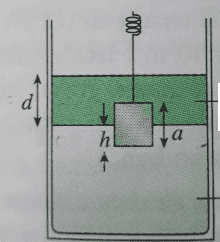# Pressure at the bottom of a cube immersed in two liquids

songoku
Homework Statement:

A cube with side a = 0.1 m is immersed in 2 liquids as shown (upper liquid is oil and lower liquid is water). The value of h is 0.02 m and the value of d is 0.2 m

Find the hydrostatic pressure at the bottom of the cube
Relevant Equations:
Hydrostatic pressure = ρ . g . hI am not sure about value of depth I need to use. What I did:

Hydrostatic pressure at bottom of cube = hydrostatic pressure by oil + hydrostatic pressure by water

= ρoil . g . d + ρwater . g . h

Is it correct I use value d = 0.2 m for depth of oil and value of h = 0.02 m for depth of water to calculate the pressure?

Thanks

Homework Helper
Is it correct I use value d = 0.2 m for depth of oil and value of h = 0.02 m for depth of water to calculate the pressure?
Yes. That is the correct approach and the correct values to use.

•songoku
songoku
Yes. That is the correct approach and the correct values to use.

Just now the teacher said my answer is wrong, h = 0.02 m is correct but it is wrong to use d = 0.2 m as the depth of oil. Instead, I should use a - h = 0.1 - 0.02 = 0.08 m as the depth of oil because that is the height of the cube in the oil.

I don't understand why I should use a - h , I think all height of oil should be included in calculating the pressure at bottom of the cube because all height of oil exerts pressure?

Thanks

Mentor
You are right and your teacher is wrong.

•songoku
songoku
Thank you very much jbriggs444 and Chestermiller

•